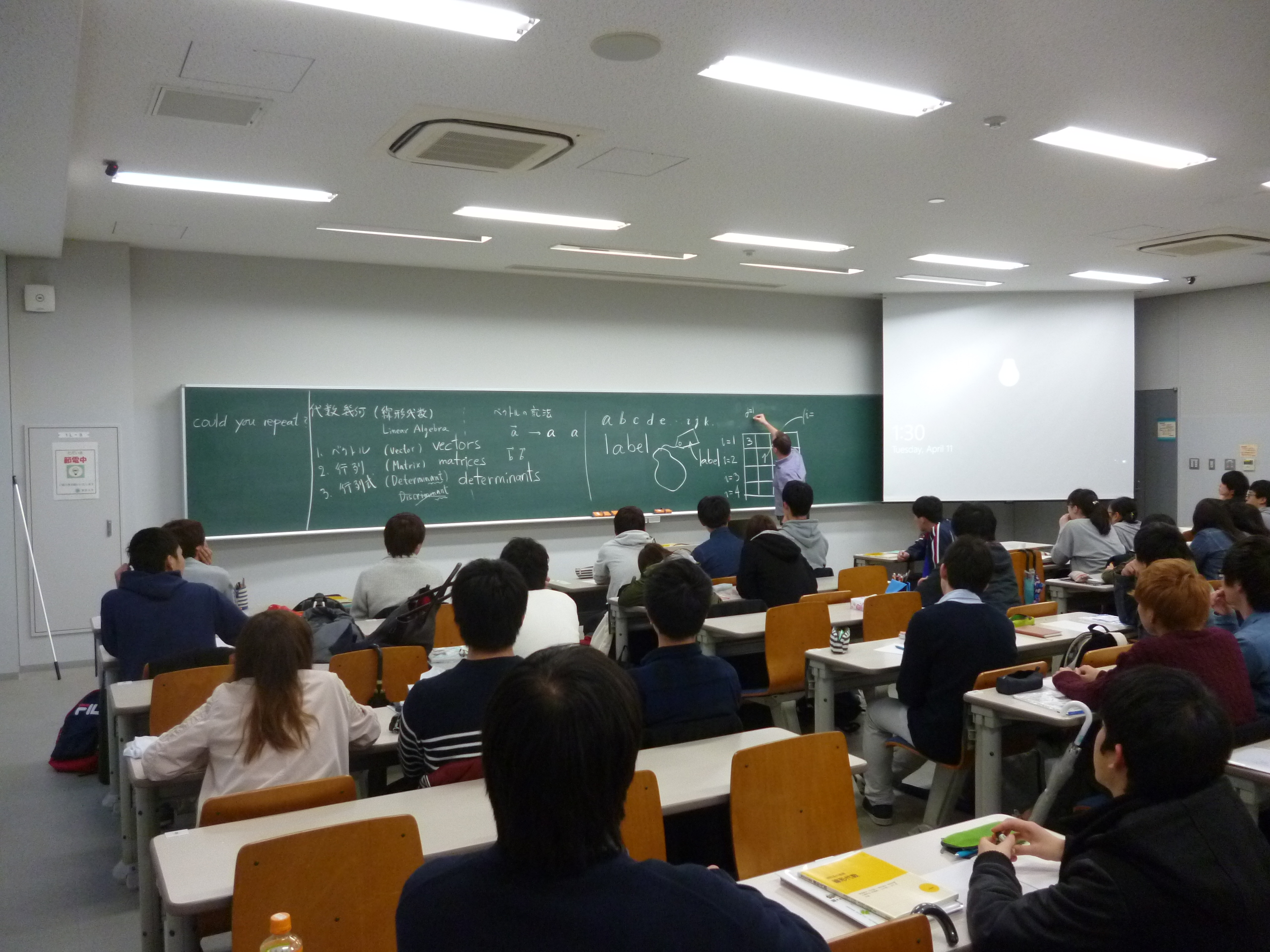### Teaching

I have lectured on more than a dozen of different topics: calculus, complex analysis, group and field theory, differential geometry, Fourier analysis, quantum physics, calculus of variations or computer-aided geometric design are some of them. This is so because here at the UASLP the maths staff is reduced to only 8 people. As you can imagine, to teach so different topics each term is somewhat stressing, and I try to write a set of notes to save some effort for the next time I am in charge of the course. Sometimes a pious student helps me transcribing them to LaTeX, sometimes I find the topic so interesting that I keep writing and eventually come up with something more or less finished.

One of my earlier duties was a course on Calculus (univariate and multivariate). The audience was a mixture of physics, mathematics and engineering students, so I had to adopt a hands-on approach. Even with this attitude, most of the students were prone to make computational errors often enough as to put at risk their final gradings, so I decided to do all the computations with a CAS. Maxima was the obvious choice for me, so I ended up writing a set of notes on Calculus with Maxima. The result is here (in Spanish).Another topic which is well-suited for a CAS-based course is 'differential equations' (and related friends, such as dynamical systems, classical mechanics and the like). Some notes working with Maxima are available here (in Spanish).

Several years ago, I wrote some notes (in Spanish) for a course in complex analysis, recalling the basics of line integrals and Green's theorem. No proofs here, only examples of calculations, but may be of use if you are in a hurry and do not remember very well how to do those integrals.

Here is a brief document (yes, in Spanish...) explaining how to study the distribution of zeros of Bessel functions with (wx)Maxima.